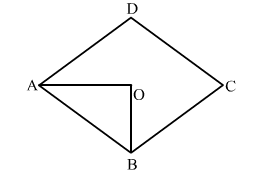# In a quadrilateral ABCD, if AO and BO are the bisectors of ∠A and ∠B respectively, ∠C = 70°

Question:

In a quadrilateral ABCD, if AO and BO are the bisectors of A and B respectively, ∠C = 70° and ∠D = 30°. Then, ∠AOB = ?
(a) 40°
(b) 50°
(c) 80°
(d) 100°

Solution:

(b) 50o

​​Explanation:C = 70o and ∠= 30o
Then A + ∠B = 360- (70 +30)o = 260o

$\therefore \frac{1}{2}(\angle A+\angle B)=\frac{1}{2}\left(260^{\circ}\right)=130^{\circ}$

In ∆​ AOB, we have:

$\angle A O B=180^{\circ}-\left[\frac{1}{2}(\angle A+\angle B)\right]$

⇒ ∠AOB​ = ​180 - 130 = 50o